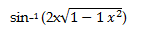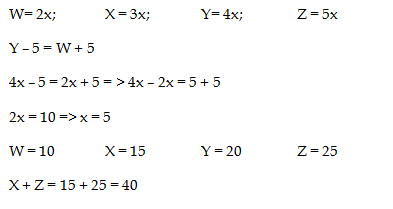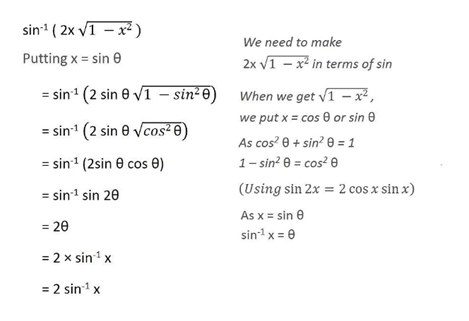# RRB ALP 2018 Practice Test Papers | Arithmetic Questions (Day-82)

Dear Aspirants, Here we have given the Important RRB ALP & Technicians Exam 2018 Practice Test Papers. Candidates those who are preparing for RRB ALP 2018 can practice these Arithmetic Questions to get more confidence to Crack RRB 2018 Examination.

[WpProQuiz 3162]

Click “Start Quiz” to attend these Questions and view Solutions

1)Find the value of x
320% of 45 + 75% of 80 = x^2 – 21

a) 16

b) 17

c) 15

d) 19

2) 5670 + 5432 + 2340 – 3450 – 2311 – 3604 = ?

a) 4087

b) 4076

c) 4077

d) 4057

3) Pipe Y can fill a tank in 6 hours and Pipe Z takes half of the time to fill the same tank. If both the pipes are open for one hour and then Pipe Z is closed. Pipe Y take how long to fill the tank alone?

a) 5 hours

b) 4 hours

c) 2 hours

d) 3 hours

4) Average ages of Ajay and Vijay is 2 : 3. After 5 years the ratio of Ajay and Suman is 4 : 3. Find the ratio of Ajay and Suman?

a) 3: 2

b) 2: 3

c) 4 : 5

d) Cannot be determine

5) W, X, Y and Z are having some chocolates in the ratio of 2: 3: 4: 5.  If Y giving 5 chocolates to W, then they have equal number of Chocolates. Find the total number chocolate having X and Z?

a) 20

b) 30

c) 40

d) 50

6) The product of two numbers is 1344.  HCF of the number is 8. Find L.C.M of these numbers

a) 148

b) 196

c) 168

d) 145

7) A Painter paints a home in certain days. If he assisted with someone he can finish the work in 4 days. If the Assistant alone can paint a home in 4 times more than that of painter, how many days Painter alone can paint a home?

a) 7 days

b) 5 days

c) 10 days

d) 15 days

8) Find the value ofa) sin -1 x

b) 2 sin -1 x

c) 0

d) Sin1 √x

9) Find the missing term

6, 7, 13, 20, 33, 53, 86, 139, 225, ?

a) 256

b) 364

c) 976

d) 876

10) 1111 + 22.22 + 111.1+ 2342.124 + 2342.45

a) 76977

b) 6987

c) 5689

d) 5929

Answers :

1). Answer: c)
320% of 45 + 75% of 80 = x^2 – 21
144 + 60 + 21 = x^2
225 = x^2
X = ± 15

2). Answer: c)

5670 + 5432 + 2340 – 3450 – 2311 – 3604 = 13442 – 9365 = 4077

3). Answer: d)

Pipe Y = 1/6 hours

Pipe Z =  1/ 3 hours

Y + Z = 1/3 hours

Working 1 hours = 1/3 – 1

Remain tank = 2/3

2/3 tank filled in 3 hours by Pipe Y

4). Answer: d)

Ajay + Vijay = 2x + 3x = 5x

Ajay + Suman = 4x – 5 + 3x – 5

We have 3 variables but two equations. We cannot get the Answer

5). Answer: c)6). Answer : c)

x

Products of two number = HCF * LCM

1344 = 8 * LCM

LCM = 168

7).Answer: B

Painter can do a work in 1/x days
Assistant can do it in 1/4x
1/x + 1/4x = 1/4
5/4x = 1/4
X = 5

Painter can alone paint the home in 5 days

8). Answer: b)

2 sin -1 x9). Answer: b)

6 + 7 = 13

7 + 13 = 20

13 + 20 = 33

20 + 33 = 53

33+ 53 = 86

53 + 86 = 139

86+ 139 =225

139 + 225 = 364

10). Answer: d

1111 + 22.22 + 111.1+ 2342.124 + 2342.45 = 5928.894 ≈ 5929

RRB ALP 2018 – “All in One” Study Materials and Practice Sets

0 0 votes
Rating0 Comments
Inline Feedbacks
View all comments